## Individual data analysis project

In :
import warnings
import numpy as np
import pandas as pd
import matplotlib.pyplot as plt

import seaborn as sns
sns.set_palette('Set3')

%matplotlib inline
warnings.filterwarnings('ignore')

from sklearn.model_selection import train_test_split, cross_val_score, GridSearchCV
from sklearn.model_selection import validation_curve, learning_curve
from sklearn.metrics import mean_squared_error


# Dataset and features description¶

Elo - one of the largest payment brands in Brazil. In the dataset we can see clients who use Elo and their transactions. We need to predict the loyalty score for each card_id.

The description of the files are

• train.csv - the training set
• test.csv - the test set
• sample_submission.csv - a sample submission file in the correct format - contains all card_ids you are expected to predict for.
• historical_transactions.csv - up to 3 months' worth of historical transactions for each card_id
• merchants.csv - additional information about all merchants / merchant_ids in the dataset.
• new_merchant_transactions.csv - two months' worth of data for each card_id containing ALL purchases that card_id made at merchant_ids that were not visited in the historical data.

The historical_transactions.csv and new_merchant_transactions.csv files contain information about each card's transactions. historical_transactions.csv contains up to 3 months' worth of transactions for every card at any of the provided merchant_ids. new_merchant_transactions.csv contains the transactions at new merchants (merchant_ids that this particular card_id has not yet visited) over a period of two months.

merchants.csv contains aggregate information for each merchant_id represented in the data set.

#### Main dataset:¶

In :
train = pd.read_csv('../../data/ELO/train.csv', parse_dates=['first_active_month'])


Out:
first_active_month card_id feature_1 feature_2 feature_3 target
0 2017-06-01 C_ID_92a2005557 5 2 1 -0.820283
1 2017-01-01 C_ID_3d0044924f 4 1 0 0.392913
2 2016-08-01 C_ID_d639edf6cd 2 2 0 0.688056
3 2017-09-01 C_ID_186d6a6901 4 3 0 0.142495
4 2017-11-01 C_ID_cdbd2c0db2 1 3 0 -0.159749
In :
# columns description

Out:
Columns Description
0 card_id Unique card identifier
1 first_active_month 'YYYY-MM', month of first purchase
2 feature_1 Anonymized card categorical feature
3 feature_2 Anonymized card categorical feature
4 feature_3 Anonymized card categorical feature
5 target Loyalty numerical score calculated 2 months af...

#### Historical Transactions:¶

In :
hist = pd.read_csv('../../data/ELO/historical_transactions.csv')

Out:
authorized_flag card_id city_id category_1 installments category_3 merchant_category_id merchant_id month_lag purchase_amount purchase_date category_2 state_id subsector_id
0 Y C_ID_4e6213e9bc 88 N 0 A 80 M_ID_e020e9b302 -8 -0.703331 2017-06-25 15:33:07 1.0 16 37
1 Y C_ID_4e6213e9bc 88 N 0 A 367 M_ID_86ec983688 -7 -0.733128 2017-07-15 12:10:45 1.0 16 16
2 Y C_ID_4e6213e9bc 88 N 0 A 80 M_ID_979ed661fc -6 -0.720386 2017-08-09 22:04:29 1.0 16 37
3 Y C_ID_4e6213e9bc 88 N 0 A 560 M_ID_e6d5ae8ea6 -5 -0.735352 2017-09-02 10:06:26 1.0 16 34
4 Y C_ID_4e6213e9bc 88 N 0 A 80 M_ID_e020e9b302 -11 -0.722865 2017-03-10 01:14:19 1.0 16 37
In :
# columns description

Out:
Columns Description
0 card_id Card identifier
1 month_lag month lag to reference date
2 purchase_date Purchase date
3 authorized_flag Y' if approved, 'N' if denied
4 category_3 anonymized category
5 installments number of installments of purchase
6 category_1 anonymized category
7 merchant_category_id Merchant category identifier (anonymized )
8 subsector_id Merchant category group identifier (anonymized )
9 merchant_id Merchant identifier (anonymized)
10 purchase_amount Normalized purchase amount
11 city_id City identifier (anonymized )
12 state_id State identifier (anonymized )
13 category_2 anonymized category

#### New merchant transactions¶

In :
transaction = pd.read_csv('../../data/ELO/new_merchant_transactions.csv')

Out:
authorized_flag card_id city_id category_1 installments category_3 merchant_category_id merchant_id month_lag purchase_amount purchase_date category_2 state_id subsector_id
0 Y C_ID_415bb3a509 107 N 1 B 307 M_ID_b0c793002c 1 -0.557574 2018-03-11 14:57:36 1.0 9 19
1 Y C_ID_415bb3a509 140 N 1 B 307 M_ID_88920c89e8 1 -0.569580 2018-03-19 18:53:37 1.0 9 19
2 Y C_ID_415bb3a509 330 N 1 B 507 M_ID_ad5237ef6b 2 -0.551037 2018-04-26 14:08:44 1.0 9 14
3 Y C_ID_415bb3a509 -1 Y 1 B 661 M_ID_9e84cda3b1 1 -0.671925 2018-03-07 09:43:21 NaN -1 8
4 Y C_ID_ef55cf8d4b -1 Y 1 B 166 M_ID_3c86fa3831 1 -0.659904 2018-03-22 21:07:53 NaN -1 29
In :
# columns description

Out:
Columns Description
0 card_id Card identifier
1 month_lag month lag to reference date
2 purchase_date Purchase date
3 authorized_flag Y' if approved, 'N' if denied
4 category_3 anonymized category
5 installments number of installments of purchase
6 category_1 anonymized category
7 merchant_category_id Merchant category identifier (anonymized )
8 subsector_id Merchant category group identifier (anonymized )
9 merchant_id Merchant identifier (anonymized)
10 purchase_amount Normalized purchase amount
11 city_id City identifier (anonymized )
12 state_id State identifier (anonymized )
13 category_2 anonymized category

# Little bit preprocessing¶

In :
train.info()

<class 'pandas.core.frame.DataFrame'>
RangeIndex: 201917 entries, 0 to 201916
Data columns (total 6 columns):
first_active_month    201917 non-null datetime64[ns]
card_id               201917 non-null object
feature_1             201917 non-null int64
feature_2             201917 non-null int64
feature_3             201917 non-null int64
target                201917 non-null float64
dtypes: datetime64[ns](1), float64(1), int64(3), object(1)
memory usage: 9.2+ MB


As features are categorical we can change type to free some memory.

In :
train['feature_1'] = train['feature_1'].astype('category')
train['feature_2'] = train['feature_2'].astype('category')
train['feature_3'] = train['feature_3'].astype('category')

test['feature_1'] = test['feature_1'].astype('category')
test['feature_2'] = test['feature_2'].astype('category')
test['feature_3'] = test['feature_3'].astype('category')

In :
train.info()

<class 'pandas.core.frame.DataFrame'>
RangeIndex: 201917 entries, 0 to 201916
Data columns (total 6 columns):
first_active_month    201917 non-null datetime64[ns]
card_id               201917 non-null object
feature_1             201917 non-null category
feature_2             201917 non-null category
feature_3             201917 non-null category
target                201917 non-null float64
dtypes: category(3), datetime64[ns](1), float64(1), object(1)
memory usage: 5.2+ MB


# Exploratory data analysis and feature engineering¶

#### Check missed data¶

In :
train.isna().sum()

Out:
first_active_month    0
card_id               0
feature_1             0
feature_2             0
feature_3             0
target                0
dtype: int64
In :
test.isna().sum()

Out:
first_active_month    1
card_id               0
feature_1             0
feature_2             0
feature_3             0
dtype: int64

#### Target column¶

Let start analys with target value

In :
fig, ax = plt.subplots(figsize = (16, 6))
plt.suptitle('Target value distribution', fontsize=24)
sns.distplot(train['target'], bins=50, ax=ax);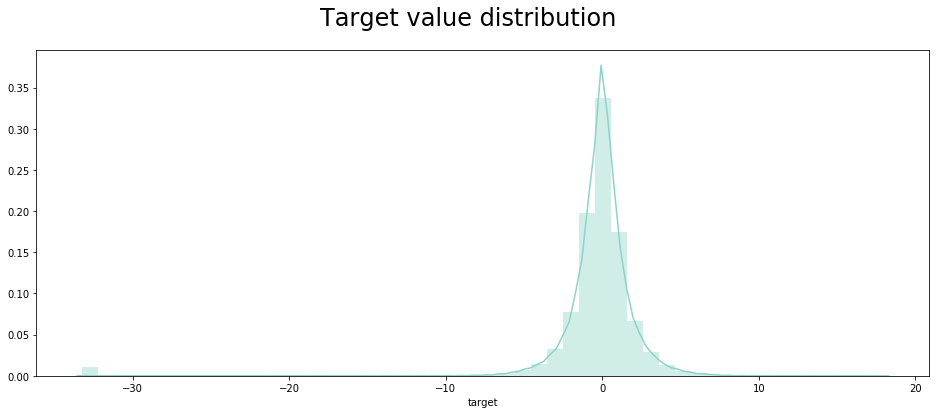We can see that some of the loyalty values are far apart (less than -30) compared to others.

In :
(train['target'] < -30).sum(), round((train['target'] < -30).sum() / train['target'].count(), 2)

Out:
(2207, 0.01)

So, there is 2207 rows (about 1% of the data), which has values different from the rest. Since the metric RMSE these rows might play an important role. So beware of them.

#### First Active Month¶

In this section, let see if there are any distribution change between train and test sets with respect to first active month of the card.

In :
fig, ax = plt.subplots(figsize = (14, 6))

first_month_count_train = train['first_active_month'].dt.date.value_counts().sort_index()
sns.barplot(first_month_count_train.index,
first_month_count_train.values,
alpha=0.8, ax=ax, color='#96CAC0')

first_month_count_test = test['first_active_month'].dt.date.value_counts().sort_index()
sns.barplot(first_month_count_test.index,
first_month_count_test.values,
alpha=0.8, ax=ax, color='#F6F6BC')

plt.xticks(rotation='vertical')
plt.xlabel('First active month', fontsize=12)
plt.ylabel('Number of cards', fontsize=12)
plt.title('First active month count')

plt.show()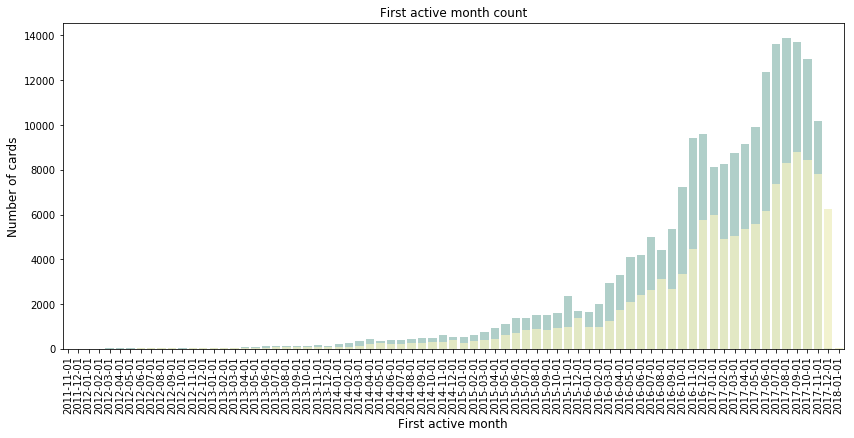Looks like the distribution is kind of similar between train and test set. So we need not really have to do time based split I think.

#### Anonymous features¶

In this section, let see if the other variables in the train dataset has good predictive power in finding the loyalty score.

In :
fig, ax = plt.subplots(1, 3, figsize = (16, 6))
plt.suptitle('Counts of categiories for features', fontsize=24)
sns.countplot(data=train, x='feature_1', ax=ax)
sns.countplot(data=train, x='feature_2', ax=ax).set(ylabel=None)
sns.countplot(data=train, x='feature_3', ax=ax).set(ylabel=None);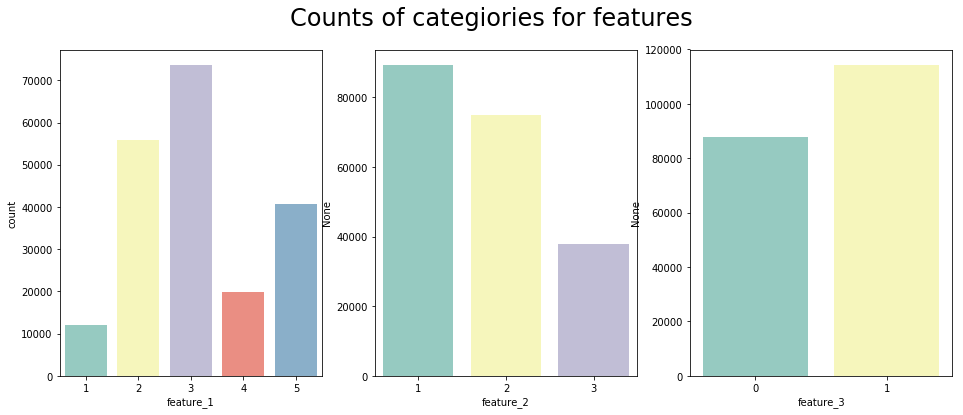In :
fig, ax = plt.subplots(1, 3, figsize=(16, 6))
plt.suptitle('Violineplots for features and target', fontsize=24)
sns.violinplot(x='feature_1', y='target', data=train, ax=ax, title='feature_1', palette='Set3')
sns.violinplot(x='feature_2', y='target', data=train, ax=ax, title='feature_2', palette='Set3')
sns.violinplot(x='feature_3', y='target', data=train, ax=ax, title='feature_3', palette='Set3');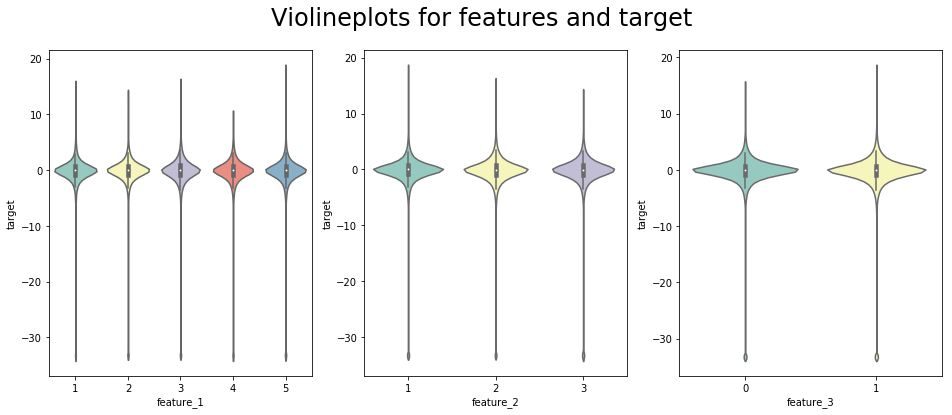To the naked eyes, the distribution of the different categories in all three features look kind of similar. May be the models are able to find something here.

Now let us make some features based on the historical transactions and merge them with train and test set.

#### Number of Historical Transactions for the card¶

In :
history_purchase_amount = hist.groupby('card_id')['purchase_amount'].size().reset_index()
history_purchase_amount.columns = ['card_id', 'history_purchase_amount']
train = pd.merge(train, history_purchase_amount, on='card_id', how='left')
test = pd.merge(test, history_purchase_amount, on='card_id', how='left')

In :
history_purchase_amount = train.groupby('history_purchase_amount')['target'].mean().sort_index()[:-50]
fig, ax = plt.subplots(figsize=(16, 6))
plt.suptitle('Loyalty score by Number of historical transactions', fontsize=24)
sns.lineplot(history_purchase_amount.index[::-1],
history_purchase_amount.values[::-1],
ax=ax);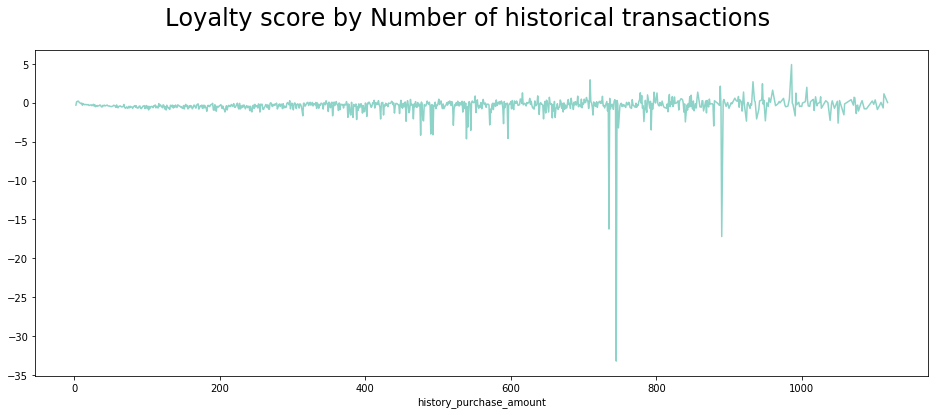Now let bin the count of historical transactions and then do some box plots to see the plots better.

In :
bins =  + [2 ** p for p in range(4, 13)]
train['binned_history_purchase_amount'] = pd.cut(train['history_purchase_amount'], bins)

plt.figure(figsize=(16, 6))
sns.boxplot(x='binned_history_purchase_amount', y='target', data=train, showfliers=False)
plt.xticks(rotation='vertical')
plt.xlabel('binned_num_hist_transactions', fontsize=12)
plt.ylabel('Loyalty score', fontsize=12)
plt.title('Distribution of binned history purchase amount', fontsize=24)
plt.show()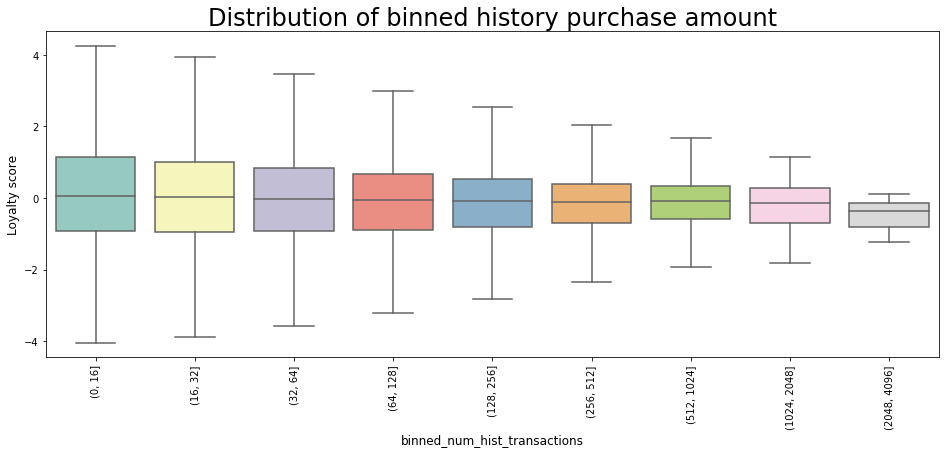#### Value of Historical Transactions¶

Check the value of the historical transactions for the cards and check the loyalty score distribution based on that.

In :
gdf = hist.groupby('card_id')['purchase_amount'].agg(['sum', 'mean', 'std', 'min', 'max']).reset_index()
gdf.columns = ['card_id',
'sum_history_purchase_amount',
'mean_history_purchase_amount',
'std_history_purchase_amount',
'min_history_purchase_amount',
'max_history_purchase_amount']
train = pd.merge(train, gdf, on='card_id', how='left')
test = pd.merge(test, gdf, on='card_id', how='left')

In :
bins = np.percentile(train['sum_history_purchase_amount'], range(0,101,10))
train['binned_sum_history_purchase_amount'] = pd.cut(train['sum_history_purchase_amount'], bins)

plt.figure(figsize=(16, 6))
sns.boxplot(x='binned_sum_history_purchase_amount', y='target', data=train, showfliers=False)
plt.xticks(rotation='vertical')
plt.xlabel('Binned sum history purchase amount', fontsize=12)
plt.ylabel('Loyalty score', fontsize=12)
plt.title('Sum of historical transaction value (binned) distribution', fontsize=24)
plt.show()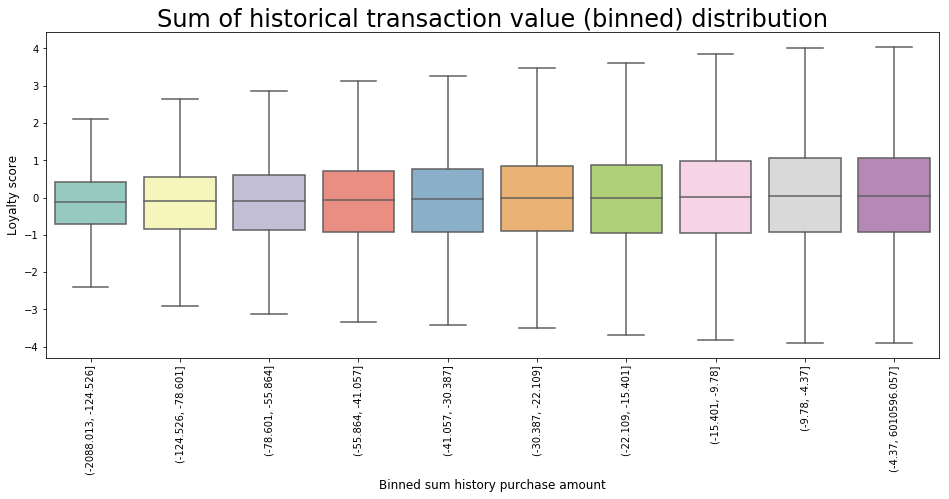As we could see, the loyalty score seem to increase with the sum of historical transaction value. This is expected. Now we can do the same plot with Mean value of historical transaction.

In :
bins = np.percentile(train['mean_history_purchase_amount'], range(0,101,10))
train['binned_mean_history_purchase_amount'] = pd.cut(train['mean_history_purchase_amount'], bins)

plt.figure(figsize=(16, 6))
sns.boxplot(x='binned_mean_history_purchase_amount', y='target', data=train, showfliers=False)
plt.xticks(rotation='vertical')
plt.xlabel('Binned Mean Historical Purchase Amount', fontsize=12)
plt.ylabel('Loyalty score', fontsize=12)
plt.title('Mean of historical transaction value (binned) distribution', fontsize=24)
plt.show()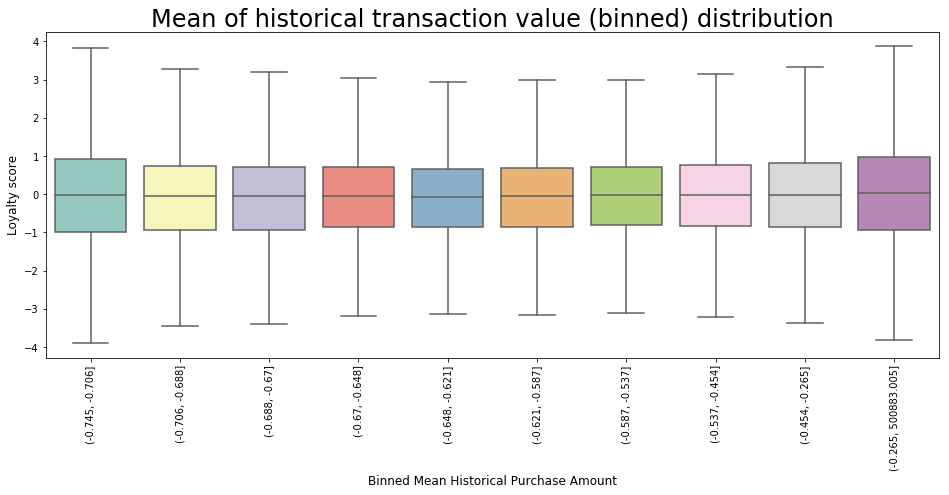#### New Merchant Transactions¶

In this section, let look at the new merchant transactions data and do some analysis

In :
gdf = transaction.groupby('card_id')['purchase_amount'].size().reset_index()
gdf.columns = ['card_id', 'transactions_count']
train = pd.merge(train, gdf, on='card_id', how='left')
test = pd.merge(test, gdf, on='card_id', how='left')

In :
bins = [0, 10, 20, 30, 40, 50, 75, 10000]
train['binned_transactions_count'] = pd.cut(train['transactions_count'], bins)

plt.figure(figsize=(16, 6))
sns.boxplot(x='binned_transactions_count', y='target', data=train, showfliers=False)
plt.xticks(rotation='vertical')
plt.xlabel('Binned transactions count', fontsize=12)
plt.ylabel('Loyalty score', fontsize=12)
plt.title('Number of new merchants transaction (binned) distribution', fontsize=24)
plt.show()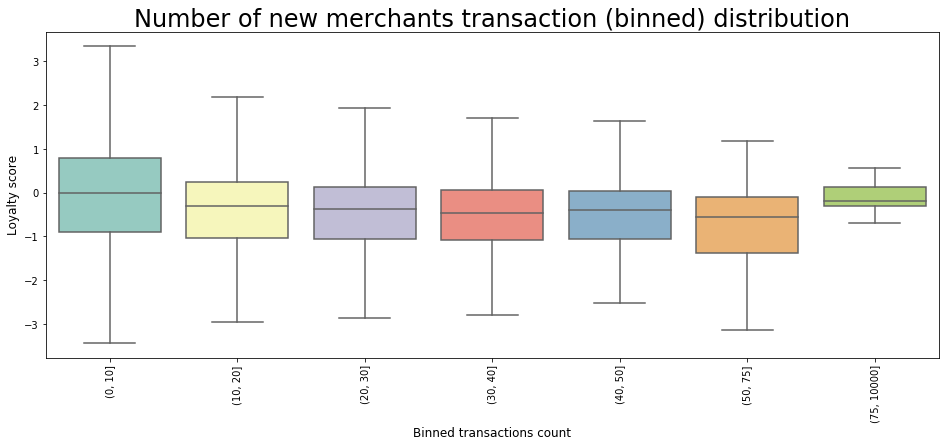Loyalty score seem to decrease as the number of new merchant transactions increases except for the last bin.

In :
gdf = transaction.groupby('card_id')['purchase_amount'].agg(['sum', 'mean', 'std', 'min', 'max']).reset_index()
gdf.columns = ['card_id',
'sum_transactions_count',
'mean_transactions_count',
'std_transactions_count',
'min_transactions_count',
'max_transactions_count']
train = pd.merge(train, gdf, on='card_id', how='left')
test = pd.merge(test, gdf, on='card_id', how='left')

In :
bins = np.nanpercentile(train['sum_transactions_count'], range(0,101,10))
train['binned_sum_transactions_count'] = pd.cut(train['sum_transactions_count'], bins)

plt.figure(figsize=(16, 6))
sns.boxplot(x='binned_sum_transactions_count', y='target', data=train, showfliers=False)
plt.xticks(rotation='vertical')
plt.xlabel('binned sum of new merchant transactions', fontsize=12)
plt.ylabel('Loyalty score', fontsize=12)
plt.title('Sum of new merchants transaction value (binned) distribution', fontsize=24)
plt.show()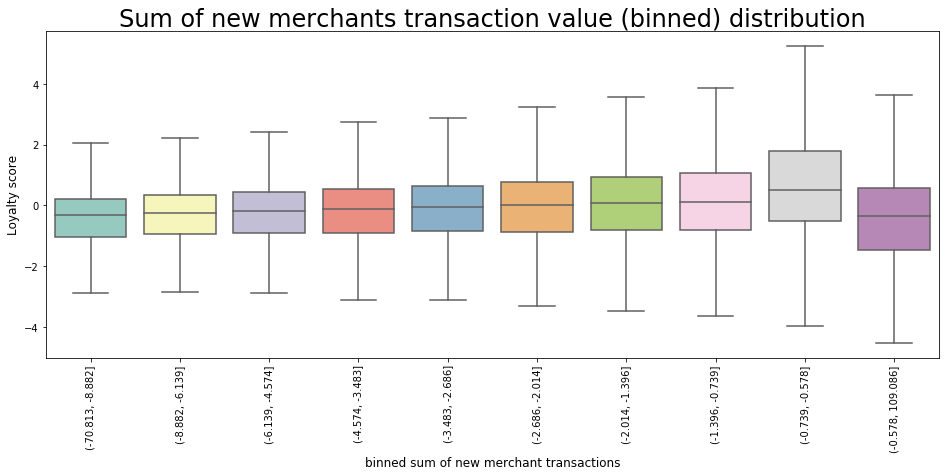Loyalty scores seem to increase with the increase in the sum of new merchant transaction values but for the last bin.

In :
bins = np.nanpercentile(train['mean_transactions_count'], range(0,101,10))
train['binned_mean_transactions_count'] = pd.cut(train['mean_transactions_count'], bins)

plt.figure(figsize=(16, 6))
sns.boxplot(x='binned_mean_transactions_count', y='target', data=train, showfliers=False)
plt.xticks(rotation='vertical')
plt.xlabel('binned mean of new merchant transactions', fontsize=12)
plt.ylabel('Loyalty score', fontsize=12)
plt.title('Mean of New merchants transaction value (binned) distribution', fontsize=24)
plt.show()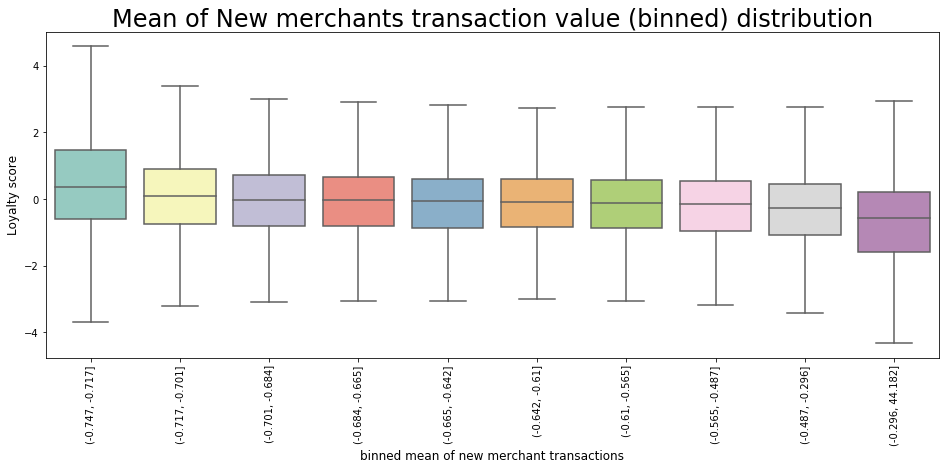# Patterns, insights, pecularities of data¶

So, according to the results of the data analysis, the following conclusions can be drawn:

• There are no gaps in the train/tets data, but detailed information provided only for the last 3 month, so we have some missed data in generated features.
• There are outliers in the target variable that require additional analysis. This could be fraud blocking, or, for example, badly filled gaps.
• Judging by the dependence of loyalty on the number of purchases, loyalty grows with a sufficiently large number of purchases (> 75), and before that usually falls. This is expected, since those who stopped at a small number of purchases, as a rule, are not satisfied with the service.

# Data preprocessing¶

1 row in test data have missed first_active_month, so lets fix it.

In :
test.loc[test['first_active_month'].isna(), 'first_active_month'] = test.loc[
(test['feature_1'] == 5) & (test['feature_2'] == 2) & (test['feature_3'] == 1),
'first_active_month'].min()


Fill in the data on card_id that do not have transactions over the past three months.

In :
cols_to_fill = [
'transactions_count', 'sum_transactions_count',
'mean_transactions_count', 'std_transactions_count',
'min_transactions_count', 'max_transactions_count',
]

train[cols_to_fill] = train[cols_to_fill].fillna(0)
test[cols_to_fill] = test[cols_to_fill].fillna(0)


Here we add common date features.

In :
max_date = train['first_active_month'].dt.date.max()
def process_main(df):
date_parts = ['year', 'weekday', 'month']
for part in date_parts:
part_col = 'first_' + part
df[part_col] = getattr(df['first_active_month'].dt, part).astype(int)

df['elapsed_time'] = (max_date - df['first_active_month'].dt.date).dt.days

return df

In :
train = process_main(train)
test = process_main(test)


# Cross-validation, hyperparameter tuning¶

#### Baseline Model¶

Let build a baseline model using the features created so far. First of all we have to split data to train and validation sets.

In :
cols_to_use = [
'feature_1', 'feature_2', 'feature_3',
'first_year', 'first_month', 'first_weekday', 'elapsed_time',
'history_purchase_amount', 'sum_history_purchase_amount',
'mean_history_purchase_amount', 'std_history_purchase_amount',
'min_history_purchase_amount', 'max_history_purchase_amount',
'transactions_count', 'sum_transactions_count',
'mean_transactions_count', 'std_transactions_count',
'min_transactions_count', 'max_transactions_count',
]

X_train, X_holdout, y_train, y_holdout = train_test_split(train[cols_to_use],
train['target'],
test_size=0.2)
X_test = test[cols_to_use]


Now that we have prepared data, we can delete raw data.

In :
del train, test, hist, transaction

In :
params = {
'learning_rate': 0.1,
'n_estimators': 100,
'subsample': 1.0,
'max_depth': 3,
'max_features': 'sqrt',
'n_iter_no_change': 5,
'validation_fraction': 0.2,
'tol': 0.00001,
'random_state': 11,
}


Fit baseline model

In :
%%time
model.fit(X_train[cols_to_use], y_train)

Wall time: 3.68 s

In :
score = mean_squared_error(y_holdout, model.predict(X_holdout))
print(f'Baseline model score: {np.sqrt(score)}')

Baseline model score: 3.7450325832910587

In :
fi = list(zip(cols_to_use, model.feature_importances_))
fi = pd.DataFrame(sorted(fi, key=lambda x: x, reverse=True), columns=['Feature', 'Importance'])

In :
plt.figure(figsize=(16, 6))
sns.barplot(x='Importance', y='Feature', data=fi, orient='h')
plt.title('Features importance', fontsize=24);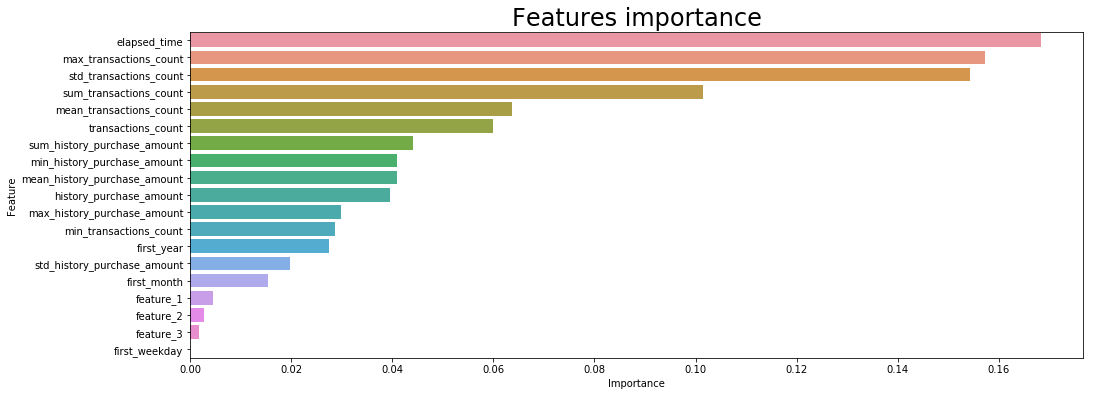# Validation and learning curves¶

Change params and tune n_estimators with validation curve.

In :
params = {
'learning_rate': 0.1,
'n_estimators': 100,
'subsample': 0.8,
'max_depth': 7,
'max_features': 'sqrt',
'n_iter_no_change': 5,
'validation_fraction': 0.2,
'tol': 0.00001,
'random_state': 11,
}

In :
def plot_validation_curve(model, X_train, y_train,
param, param_range, cv=3,
scoring='neg_mean_squared_error'):
train_scores, test_scores = validation_curve(
model, X_train, y_train, cv=cv,
param_name=param, param_range=param_range,
scoring=scoring, n_jobs=-1
)
train_scores_mean = np.mean(train_scores, axis=1)
train_scores_std = np.std(train_scores, axis=1)
test_scores_mean = np.mean(test_scores, axis=1)
test_scores_std = np.std(test_scores, axis=1)

plt.figure(figsize=(16, 6))
plt.title('Validation Curve')
plt.xlabel('n_estimators')
plt.ylabel('Score')

plt.semilogx(param_range, train_scores_mean, label='Training score',
color='darkorange', lw=2)
plt.fill_between(param_range, train_scores_mean - train_scores_std,
train_scores_mean + train_scores_std, alpha=0.2,
color='darkorange', lw=2)
plt.semilogx(param_range, test_scores_mean, label='Cross-validation score',
color='navy', lw=2)
plt.fill_between(param_range, test_scores_mean - test_scores_std,
test_scores_mean + test_scores_std, alpha=0.2,
color='navy', lw=2)
plt.legend(loc='best')
plt.show()

In :
%%time
X_train[cols_to_use], y_train,
param='n_estimators',
param_range=[10 ** x for x in range(1, 6)])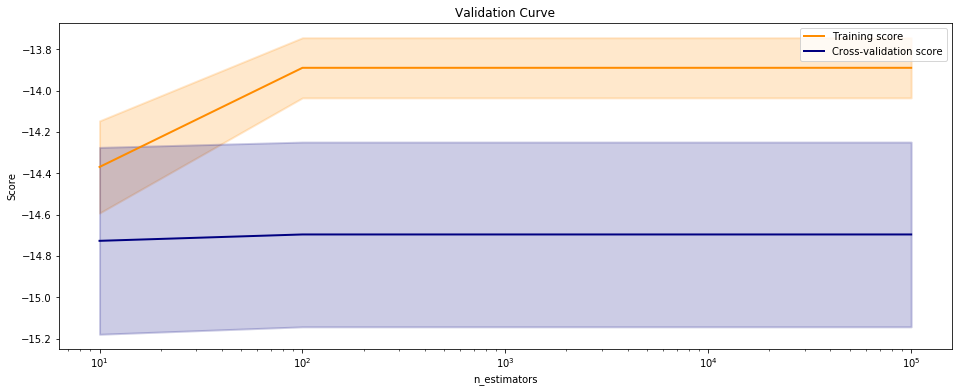Wall time: 27 s


This validation curve poses two possibilities: first, that we do not have the correct param_range to find the best n_estimators and need to expand our search to larger values. The second is that other hyperparameters (such as learning_rate or max_depth, or even subsample) may have more influence on the default model than n_estimators by itself does. Although validation curves can give us some intuition about the performance of a model to a single hyperparameter, grid search is required to understand the performance of a model with respect to multiple hyperparameters.

In :
def plot_learning_curve(model, X_train, y_train, cv=3,
train_sizes=None, scoring='neg_mean_squared_error',
random_state=11):
if not train_sizes:
train_sizes = np.linspace(.1, 1.0, 8)

train_sizes, train_scores, test_scores = learning_curve(
model, X_train, y_train, cv=cv,
train_sizes=train_sizes,
scoring=scoring,
random_state=random_state,
n_jobs=-1
)

train_scores_mean = np.mean(train_scores, axis=1)
train_scores_std = np.std(train_scores, axis=1)
test_scores_mean = np.mean(test_scores, axis=1)
test_scores_std = np.std(test_scores, axis=1)

plt.figure(figsize=(16, 6))
plt.title('Learning curve')
plt.xlabel('Training examples')
plt.ylabel('Score')
plt.grid()
plt.fill_between(train_sizes, train_scores_mean - train_scores_std,
train_scores_mean + train_scores_std, alpha=0.1,
color='r')
plt.fill_between(train_sizes, test_scores_mean - test_scores_std,
test_scores_mean + test_scores_std, alpha=0.1, color='g')
plt.plot(train_sizes, train_scores_mean, 'o-', color='r',
label='Training score')
plt.plot(train_sizes, test_scores_mean, 'o-', color='g',
label='Cross-validation score')

plt.legend(loc='best')
plt.show()

In :
%%time
plot_learning_curve(gbm, X_train[cols_to_use], y_train)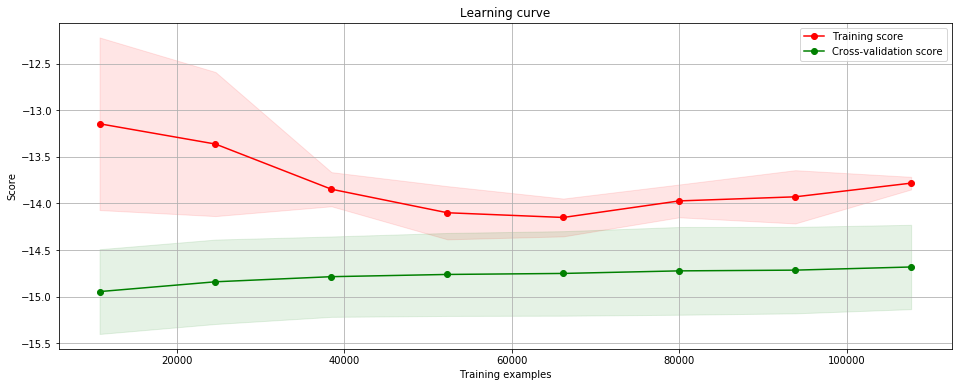Wall time: 16.9 s


This learning curve shows high test variability and a low score. We can see that the training and test scores have not yet converged, so potentially this model would benefit from more training data. Finally, this model does not suffer from error due to variance (the CV scores for the test data are more variable than for training data) so it is possible that the model is underfitting.

# Prediction for hold-out and test samples¶

In :
%%time
new_params = params
new_params['n_iter_no_change'] = None
new_params['n_estimators'] = 100
model.fit(X_train[cols_to_use], y_train)

Wall time: 5.89 s

In :
score = mean_squared_error(y_holdout, model.predict(X_holdout))
print(f'Final model score: {np.sqrt(score)}')

Final model score: 3.7444562556838603

In :
submission = pd.read_csv('../../data/ELO/sample_submission.csv')
submission['target'] = model.predict(X_test)
submission.to_csv('submit.csv', index=False)


# Metrics description¶

Predictions are scored on the root mean squared error. RMSE defined as: $$RMSE = \sqrt{ \frac{1}{n} \sum_{i=1}^n (y_i - \hat{y}_i) ^ 2 }$$ where $\hat{y}$ is the predicted loaylty score for each card_id, and $y$ is the actual loyalty score assigned to a card_id.

The RMSE is the square root of the variance of the residuals. It indicates the absolute fit of the model to the data–how close the observed data points are to the model’s predicted values. RMSE is a good measure of how accurately the model predicts the response, and it is the most important criterion for fit if the main purpose of the model is prediction.

# Model evaluation¶

The result - a fairly accurate model (the middle of the leaderboard) and having a small variance.

# Conclusions¶

To sum up, we have the model far away from perfect predictions and there is plenty room for improvement here.

• First, it would be much better fine tune parameters (frankly, I stoped wait the end of GridSearch after second night).
• Second, creating more informative features and try other models (e.g. xgboost, LightGBM, CatBoost).

So, the time has finish. Thank you for your attentions!